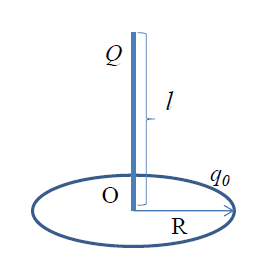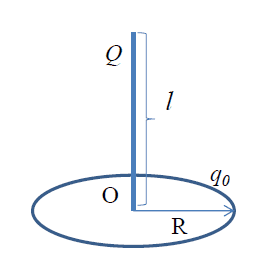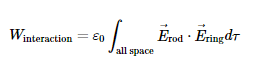# Electrostatic Interaction Energy of a Rod and a Ring

## Homework Statement

[/B]
Thin rod of length $l$ is placed with one of its ends at the center $O$ of the (thin circular )ring of radius R as shown (Figure 1), perpendicular to the plane of the ring. The rod is charged with total charge Q that is distrubted along the rod's length with linear charge density linearly proportional to the distance from the bottom end of the rod. The ring is uniformly charged with total charge $q_0$. Find the electrostatic interaction energy between the rod and the ring.

(Figure 1)## Homework Equations

(Definition of Interaction Energy)[/B]

$$W_{\text{interaction}}=\varepsilon_0\int_{\text{all space}}\vec{E}_{\text{rod}}\cdot\vec{E}_{\text{ring}}d\tau$$

(Interaction Energy of two point charges qa and qb)

$$W_{\text{interaction}} = \frac{q_a q_b}{4\pi\varepsilon_0r^2}$$

I'd imagine that finding expressions for the field of these two objects would be very cumbersome because there is little to no symmetry to exploit. We could find expressions for these fields easily if we limited ourselves to the surface of the ring or the axis of the rod, but the definition of interaction energy requires that we have expressions for field that apply to all space.

Instead I'd like to treat the system as a collection of point charges and appeal to the second equation above.

## The Attempt at a Solution

[/B]
$$dq_{rod} = \frac{2Qz}{l^2} dz$$

where dz is a differential element of length for the rod, notice that if we integrate dqrod from $0$ to $l$ we get the total charge of Q

$$dq_{ring} = \frac{q_0 dc}{2 \pi R}$$

where dc is the differential element of length(/circumference) for the ring

The interaction between a differential element of length for the rod and a differential element of length for the thin circular wire is

$$d\zeta = \frac{dq_{rod} dq_{ring}}{4\pi\varepsilon_0 (R^2 + z^2)}$$

plugging in the expression for dqrod and dqring we get

$$d\zeta = \frac{q_0 Q z dc dz}{4\pi^2\varepsilon_0 R l^2 (R^2 + z^2)}$$

If we (double) integrate this expression over ##0 \leq c \leq 2piR## and ##0 \leq z \leq l## then we should get the total interaction energy between the ring and the rod

$$\int_{z=0}^l \int_{c=0}^{2 \pi R} \frac{q_0 Q z dc dz}{4\pi^2\varepsilon_0 R l^2 (R^2 + z^2)}, dc\, dz$$

$$= \frac{q_0 Q}{2\pi\varepsilon_0l^2} \int_{z=0}^l \frac{z}{R^2 + z^2} \, dz$$

$$= \frac{q_0 Q}{4\pi\varepsilon_0l^2} \left (\ln{|R^2 +z^2|}) \right|_0^l$$

$$\zeta = \frac{q_0 Q}{4\pi\varepsilon_0 l^2} \ln{\left|\frac{R^2 + l^2}{R^2}\right|}$$ (TOTAL INTERACTION ENERGY BETWEEN ROD AND RING)

My friend contends that I am wrong because I applied the product rule incorrectly to dqringdqrod. He had a similar problem for homework about 2 years ago and he did it using the expression for fields which to me is cumbersome at best and impossible at worst. In fact he posted the same question in this section around that time https://www.physicsforums.com/threa...-energy-between-a-charge-rod-and-ring.770828/.

This is my first time posting as well as my first time using Latex so I apologize if I have unknowingly broken any rules and for formatting errors. I know my username is immature; I made it a few months ago before upper level physics forced me to grow up.

I look forward to participating in this community and I would like to say thank you in advance for any help and comments.

BvU
Homework Helper
Hello PhDNut,Impressive amount of excellent ##\LaTeX##, certainly for a first post !

You have two expressions, both making things difficult. Isn't there a way to exploit something like rewriting the second expression to ##W = \int E dq \ ## effectively, using e.g. the E field from the ring ?

That also deals with
little to no symmetry to exploit

TSny
Homework Helper
Gold Member
$$W_{\text{interaction}} = \frac{q_a q_b}{4\pi\varepsilon_0r^2}$$
Is the power of 2 correct in the denominator?

My friend contends that I am wrong because I applied the product rule incorrectly to dqringdqrod. He had a similar problem for homework about 2 years ago and he did it using the expression for fields which to me is cumbersome at best and impossible at worst. In fact he posted the same question in this section around that time https://www.physicsforums.com/threa...-energy-between-a-charge-rod-and-ring.770828/.
This is not a good approach! (as your friend realized in his/her final comment in that thread)

•PhDeezNutz
nrqed
Homework Helper
Gold Member

## Homework Statement

[/B]
Thin rod of length $l$ is placed with one of its ends at the center $O$ of the (thin circular )ring of radius R as shown (Figure 1), perpendicular to the plane of the ring. The rod is charged with total charge Q that is distrubted along the rod's length with linear charge density linearly proportional to the distance from the bottom end of the rod. The ring is uniformly charged with total charge $q_0$. Find the electrostatic interaction energy between the rod and the ring.

(Figure 1)## Homework Equations

(Definition of Interaction Energy)[/B]

$$W_{\text{interaction}}=\varepsilon_0\int_{\text{all space}}\vec{E}_{\text{rod}}\cdot\vec{E}_{\text{ring}}d\tau$$

(Interaction Energy of two point charges qa and qb)

$$W_{\text{interaction}} = \frac{q_a q_b}{4\pi\varepsilon_0r^2}$$

Note that the first expression is incorrect, (as TSNY pointed out) they do not even have the correct dimensions. But modulo this mistake, your approach looks ok.

Last edited:
•PhDeezNutz
TSny
Homework Helper
Gold MemberI think this one is correct. The integral is a volume integral over all space.

nrqed
Homework Helper
Gold Member
View attachment 101515
I think this one is correct. The integral is a volume integral over all space.
Ah yes, you are right. My mistake. I incorrectly read it as having the ## \epsilon_0## in the denominator (I am too used to see it there, I guess). Thanks for the correction.

Hello PhDNut,Impressive amount of excellent ##\LaTeX##, certainly for a first post !

You have two expressions, both making things difficult. Isn't there a way to exploit something like rewriting the second expression to ##W = \int E dq \ ## effectively, using e.g. the E field from the ring ?

That also deals with

Thanks for the replies everyone, I redid the problem using BvU's "field strategy" and then I redid the problem using the correct formula for interaction energy of a point charge. However I got two different answers which is unsettling because they both seem like sound approaches to me. Hopefully my mistakes are just mathematical

First I'll show my work for BvU's strategy

BvU's "Field Approach"

Use the field of the ring to find the interaction enrgy between each differential element of charge on the rod and the entire ring

$$\vec E_{ring} = \frac {1} {4\pi\varepsilon_0} \frac{q_0 z} {(R^2 + z^2)^{3/2}}$$

$$dq_{rod} = \frac {2Q}{l^2} z dz$$

$$W = \int E_{ring}dq_{rod}, = \frac {q_0 Q} {2\pi\varepsilon_0 l^2} \int_{z=0}^l \frac {z^2} {(R^2 + z^2)^{3/2}} dz$$

$$= \frac {q_0 Q} {2\pi\varepsilon_0 l^2} \left (\ln {\sqrt {R^2+z^2} +z} - \frac {z}{\sqrt {z^2 + R^2}} \right|_{z=0}^l$$

$$W = \frac {q_0 Q} {2\pi\varepsilon_0 l^2} \left[ \ln\left({\frac{\sqrt {R^2 + l^2} + l} {R}}\right) - \frac{l}{\sqrt{l^2 + R^2}} \right]$$

TSny
Homework Helper
Gold Member
$$W = \int E_{ring}dq_{rod}$$
Electric field multiplied by charge has the dimensions of force. But you want energy.

Your original calculation was very good except for using the wrong power of r in the denominator for the potential energy of two point charges.

Is the power of 2 correct in the denominator?

This is not a good approach! (as your friend realized in his/her final comment in that thread)

Note that the first expression is incorrect, (as TSNY pointed out) they do not even have the correct dimensions. But modulo this mistake, your approach looks ok.

Incorporating TSny's and nrqed's correction to my "point charge approach"

Alright time to do this problem with the correct expression for interaction energy of two point charges......let's do this............

(Interaction Energy of two point charges qa and qb)

$$W_{\text{interaction}} = \frac{q_a q_b}{4\pi\varepsilon_0 r}$$

$$dq_{rod} = \frac{2Qz}{l^2} dz$$

where dz is a differential element of length for the rod, notice that if we integrate dqrod from $0$ to $l$ we get the total charge of Q

$$dq_{ring} = \frac{q_0 dc}{2 \pi R}$$

where dc is the differential element of length(/circumference) for the ring

The interaction between a differential element of length for the rod and a differential element of length for the thin circular wire is

$$dW_{interact} = \frac{dq_{rod} dq_{ring}}{4\pi\varepsilon_0 \sqrt {R^2 + z^2}}$$

plugging in the expression for dqrod and dqring we get

$$dW_{interact} = \frac{q_0 Q z dc dz}{4\pi^2\varepsilon_0 R l^2 \sqrt{R^2 + z^2}}$$

If we (double) integrate this expression over ##0 \leq c \leq 2piR## and ##0 \leq z \leq l## then we should get the total interaction energy between the ring and the rod

$$\int_{z=0}^l \int_{c=0}^{2 \pi R} \frac{q_0 Q z dc dz}{4\pi^2\varepsilon_0 R l^2 \sqrt{R^2 + z^2}}, dc\, dz$$

$$= \frac{q_0 Q}{2\pi\varepsilon_0l^2} \int_{z=0}^l \frac{z}{\sqrt{R^2 + z^2}} \, dz$$

$$=\frac{q_0 Q}{4\pi\varepsilon_0l^2} \left. \sqrt{z^2 + R^2}\right|_0^l$$

$$W_{interaction} = \frac{q_0 Q}{4\pi\varepsilon_0 l^2} \left( \sqrt{R^2 + l^2} - R \right)$$ (TOTAL INTERACTION ENERGY BETWEEN ROD AND RING)

TSny
Homework Helper
Gold Member
$$dW_{interact} = \frac{dq_{rod} dq_{ring}}{4\pi\varepsilon_0 \sqrt {R^2 + z^2}}$$.
...................

$$W_{interaction} = \frac{q_0 Q}{4\pi\varepsilon_0 l^2} \left( \sqrt{R^2 + l^2} - R \right)$$ (TOTAL INTERACTION ENERGY BETWEEN ROD AND RING)
That looks right. I didn't check every step, but your setup looks good and you are getting the same answer I got using the same method.

Wait. I get a factor of 2 in the denominator instead of a factor of 4 in the final answer. Check your numerical factors when you integrate ##z/ \sqrt{R^2+z^2}##

Electric field multiplied by charge has the dimensions of force. But you want energy.

Your original calculation was very good except for using the wrong power of r in the denominator for the potential energy of two point charges.

Yeah that was unsettling to me as well. I think BvU meant to say

$$\int {Vdq}$$

Gonna try this when I get home.

That looks right. I didn't check every step, but your setup looks good and you are getting the same answer I got using the same method.

Wait. I get a factor of 2 in the denominator instead of a factor of 4 in the final answer. Check your numerical factors when you integrate ##z/ \sqrt{R^2+z^2}##

Yup. I did it again more carefully and got the same answer as you.

BvU
Homework Helper
Electric field multiplied by charge has the dimensions of force. But you want energy.

Your original calculation was very good except for using the wrong power of r in the denominator for the potential energy of two point charges.Sorry to PhD for missing that dimensional misfit and thanks to TSny for putting us right !

•PhDeezNutzSorry to PhD for missing that dimensional misfit and thanks to TSny for putting us right !

No worries. I assume you meant ##\int V_{ring}(z)dq_{rod}##. I tried this approach as well and was able to get the same answer as TSny. I would say that your approach is better than mine because it fully exploits symmetry.